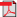# Search Results

1. P. Lassila and J. Virtamo, Nearly Optimal Importance Sampling for Monte Carlo Simulation of Loss Systems, COST257, TD(00), 2000(bib)
Abstract: In this paper we consider the problem of estimating blocking probabilities in the multiservice loss system via simulation, applying the static Monte Carlo method with importance sampling. Earlier approaches to this problem include the use of either a single exponentially twisted version of the steady state distribution of the system or a composite of individual exponentially twisted distributions. Here, a different approach is introduced, where the original estimation problem is first decomposed into independent simpler sub-problems, each roughly corresponding to estimating the blocking probability contribution from a single link. Then two importance sampling distributions are presented, which very closely approximate the ideal importance sampling distribution for each sub-problem. In both methods, the idea is to try to generate samples directly into the blocking state region. The difference between the methods is that the first method, the inverse convolution method, achieves this exactly, while the second one, using a fitted Gaussian distribution, only approximately. The inverse convolution algorithm, however, has a higher memory requirement. Finally, a dynamic control algorithm is given for optimally allocating the samples between different sub-problems. The numerical results demonstrate that the variance reduction obtained with the methods, especially with the inverse convolution method, is truly remarkable, between 400 and 500 000 in the considered examples.